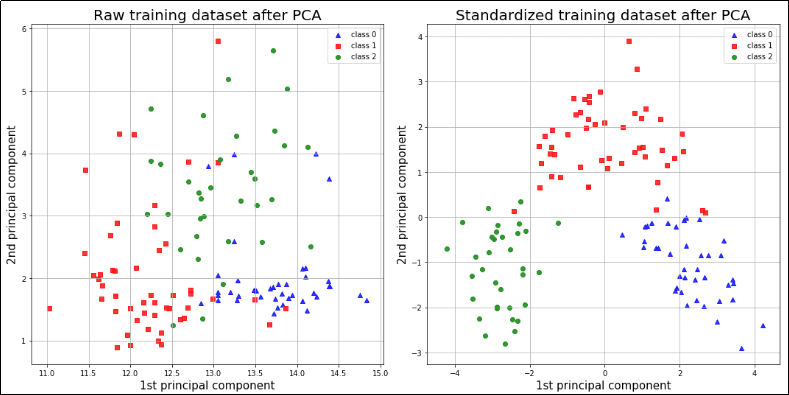Feature Scaling: Quick Introduction and Examples using Scikit-learn

# Feature Scaling: Quick Introduction and Examples using Scikit-learn

Last updated:

WIP Alert This is a work in progress. Current information is correct but more content may be added in the future.

WHY:

• Feature scaling helps avoid problems when some features are much larger (in absolute value) than other features.

• You need it for all techniques that use distances in any way (i.e. which are scale-variant) such as:

• SVM (Support Vector Machines)

• k-Nearest Neighbours

• PCA (Principal Component Analysis)

• You must perform feature scaling in any technique that uses SGD (Stochastic Gradient Descent), such as:

• Neural networks
• Logistic Regresssion*Scale-variant methods like PCA exhibit DRASTICALLY different results depending on whether
they were applied on standardized or raw features. *

Remember to scale train/test data separately, otherwise you're leaking data!

## Simple Feature Recaling

Also called min-max Scaling

WHAT:

• Subtract the minimum value and divide by the total feature range (max-min).

• Transformed features now lie between 0 and 1

WHEN TO USE:

• TODO

NOTES:

• Heavily influenced by outliers.

EXAMPLE:

from sklearn.preprocessing import MinMaxScaler

scaler = MinMaxScaler()
scaler.fit(X_train)

X_train = scaler.transform(X_train)
X_test = scaler.transform(X_test)


## Standardization

WHAT:

• Subtract the mean and divide by the standard deviation.

WHEN TO USE:

• When your data has many outliers.

• When you need your data to have zero mean.

EXAMPLE:

from sklearn.preprocessing import StandardScaler

scaler = StandardScaler()
scaler.fit(X_train)

X_train = scaler.transform(X_train)
X_test = scaler.transform(X_test)


## Normalization

WHAT:

• Scale every feature vector so that it has norm = 1.

• Usually you'll use L2 (euclidean) norm but you can also use others.

WHEN TO USE:

• When you are going to apply methods such as dot products on the feature vectors.

NOTES:

• Because this transformation does not depend on other points in your dataset, calling .fit() has no effect.

EXAMPLE:

from sklearn.preprocessing import Normalizer

normalizer = Normalizer()

# this does nothing because this method doesn't 'train' on your data
normalizer.fit(X_train)

X_train = normalizer.transform(X_train)
X_test = normalizer.transform(X_test)


## Robust Scaling

TODO


## TODO

CHECK THE VALIDITY OF THIS INFO

source: https://medium.com/towards-data-science/top-6-errors-novice-machine-learning-engineers-make-e82273d394db